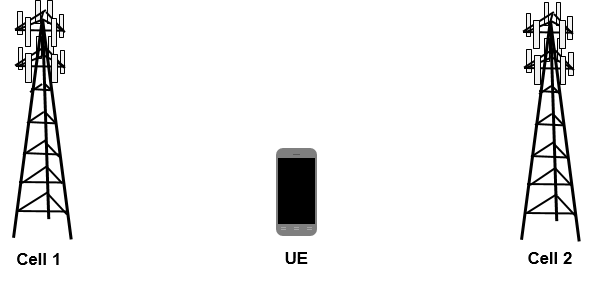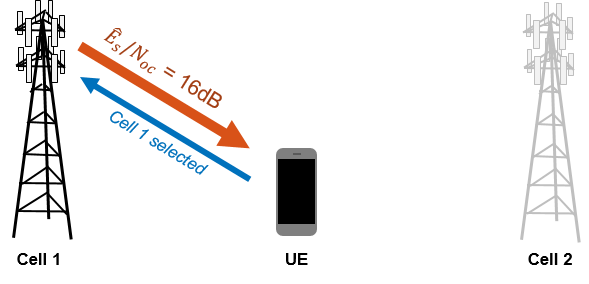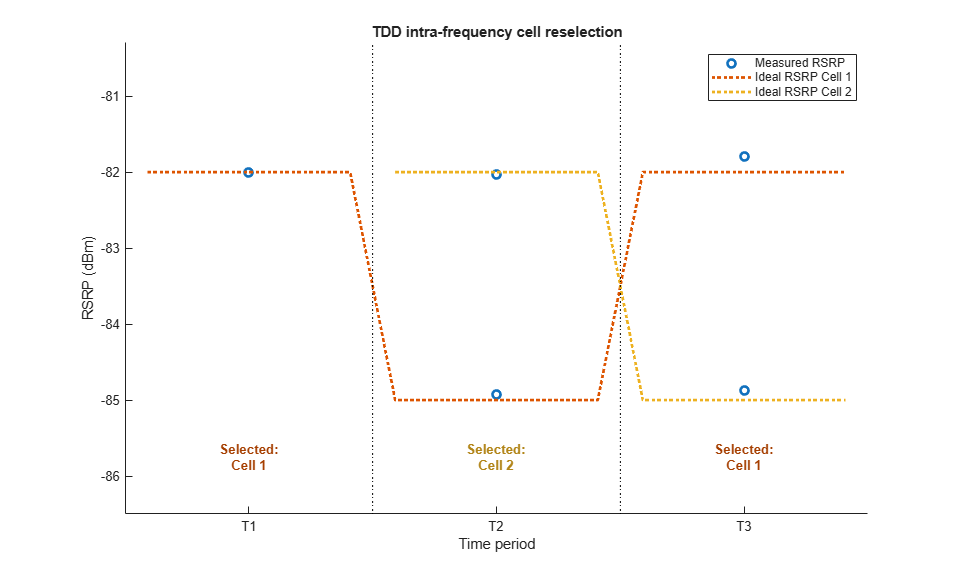# Reference Signal Measurements (RSRP, RSSI, RSRQ) for Cell Reselection

In the LTE system, a UE must detect and monitor the presence of multiple cells and perform cell reselection to ensure that it is "camped" on the most suitable cell. A UE "camped" on a particular cell will monitor the System Information and Paging of that cell, but it must continue to monitor the quality and strength of other cells to determine if cell reselection is required.

In this example, the cell reselection test environment described in TS 36.133 Annex A.4.2.2.1 [ 1 ] is configured. Cell search is then performed to determine the detected cells. Reference Signal (RS) measurements are made:

• Reference Signal Received Power (RSRP)

• Reference Signal Received Quality (RSRQ)

Finally the RSRP is used as the criterion for cell reselection.

### Introduction

The purpose of the test in TS 36.133 Annex A.4.2.2.1 [ 1 ] is to verify that the requirements for TDD to TDD intra-frequency cell reselection are met. The test environment consists of a single TDD carrier, a single UE and two cells (Cell 1 and Cell 2) as shown in the diagram below:The test then defines three successive time periods T1...T3, during which Cell 1 and Cell 2 have different power levels. In each of the time periods, the UE must select the correct cell based on the cell reselection criteria defined in TS 36.304 Section 5.2 [ 2 ]. Those criteria involve the measured RSRP and RSRQ, minimum required RSRP and RSRQ levels, and various offsets. In this example, a simplified reselection procedure is used, where the cell with the highest RSRP is selected.

The power levels of each cell and the expected behavior of the UE in each time period are as follows:

In time period T1, only Cell 1 is active and Cell 2 is powered off. The UE should select Cell 1:In time period T2, Cell 2 is powered on and the power of Cell 1 is decreased. The UE should select Cell 2:In time period T3, the power of Cell 2 is decreased and the power of Cell 1 is increased. The UE should select Cell 1:This example will show how to use the LTE Toolbox™ to configure the test environment in TS 36.133 Annex A.4.2.2.1 [ 1 ], perform cell search to detect the cells which are present, and finally make RSRP measurements in order to perform cell reselection.

### Signal Power Setup

The signal powers (in dB) for both cells in each time period are set up according to TS 36.133 Table A.4.2.2.1-2 [ 1 ]. The signal powers are vectors, where each element gives the signal power for each of the three time periods of the test.

SINRdB1 = [ 16 13 16]; % Es/Noc for Cell 1 SINRdB2 = [-Inf 16 13]; % Es/Noc for Cell 2 

### Noise Power Setup

The noise power (in dBm) is set up according to TS 36.133 Table A.4.2.2.1-2 [ 1 ]. The linear noise power is then calculated and will be used later to configure the AWGN added in the test.

NocdBm = -98; % dBm/15kHz average power spectral density NocdBW = NocdBm-30; % Noc in dBW/15kHz Noc = 10^(NocdBW/10); % linear Noc 

### Cell 1 Configuration

The function lteRMCDL, which creates a Reference Measurement Channel (RMC) configuration, is used to create a complete eNodeB configuration for Cell 1. The RMC used is RMC R.7, which has the required bandwidth of 10MHz as specified in TS 36.133 Table A.4.2.2.1-1 [ 1 ]. The TDD uplink-downlink configuration and special subframe configuration for the test are also specified in that table. Orthogonal Channel Noise Generation (OCNG) is enabled and an arbitrarily-chosen cell identity is set.

cell1 = lteRMCDL('R.7','TDD'); cell1.TDDConfig = 1; cell1.SSC = 6; cell1.OCNGPDCCHEnable = 'On'; cell1.OCNGPDSCHEnable = 'On'; cell1.NCellID = 101; 

### Cell 2 Configuration

The configuration of Cell 2 is identical to that of Cell 1 except a different cell identity is used.

cell2 = cell1; cell2.NCellID = 313; 

### Cell Search Configuration

A structure searchalg is created, which will be used to configure the operation of the lteCellSearch function used to detect cells. When detecting multiple cells, this function ranks the cells according to the peak magnitude of the correlations used to detect PSS and SSS, rather than RSRP. Therefore MaxCellCount, the number of cells to detect, is set to 3 as the strongest two cells in terms of RSRP (expected to be Cell 1 and Cell 2) are not necessarily the strongest two cells detected by the lteCellSearch function. The SSS detection method is set to 'PostFFT', where SSS detection is performed in the frequency domain, with the OFDM demodulation synchronized using the timing estimate from PSS detection.

searchalg.MaxCellCount = 3; searchalg.SSSDetection = 'PostFFT'; 

### Simulation Loop for Test Time Periods

The simulation is run in a loop for the three time periods T1...T3 defined in the test. The processing steps for each time period are as follows:

• Cell 1 is transmitted at the specified power level for the time period

• Cell 2 is transmitted at the specified power level for the time period, and the timing offset between cells specified in TS 36.133 Table A.4.2.2.1-1 [ 1 ] is applied

• An AWGN waveform is created at the specified power level for the test, and the Cell 1, Cell 2 and AWGN waveforms are added together to model the received waveform at the UE

• Cell search is performed on the received waveform

• For each detected cell, the received waveform is synchronized, OFDM demodulated and the RSRP is measured (using the function hRSMeasurements) and the detected cell identities are listed in order of decreasing RSRP

• The selected cell (Cell 1 or Cell 2) is determined by selecting the cell identity which has the highest measured RSRP

A number of values are recorded at the MATLAB® Command Window for each time period:

• For Cell 1 and Cell 2: the cell identity, SINR (/), SNR (/) and ideal RSRP (measured from the transmitted waveforms)

• For each detected cell at the receiver: the cell identity and the measured RSRP (measured from the received waveform)

• The selected cell (and its cell identity)

Note that a number of other physical layer parameters such as the cyclic prefix length and duplex mode are assumed to be known and are assumed to be equal for each eNodeB. See the Cell Search, MIB and SIB1 Recovery example for more information on detecting these parameters.

nTimePeriods = 3; txRSRPs = -inf(nTimePeriods,2); rxRSRPs = -inf(nTimePeriods,searchalg.MaxCellCount); detectedCells = zeros(nTimePeriods,1); rng('default'); separator = repmat('-',1,44); % For each time period: for T = 1:nTimePeriods fprintf('\n%s\n Time period T%d\n%s\n\n',separator,T,separator); fprintf(' tx: Cell 1 Cell 2\n'); fprintf(' NCellID: %7d %7d\n',cell1.NCellID,cell2.NCellID); % Cell 1 transmission. SINR1 = 10^(SINRdB1(min(T,end))/10); % linear Es/Noc Es1 = SINR1*Noc; % linear Es per RE [txcell1,~,info] = lteRMCDLTool(cell1,randi([0 1],1000,1)); txcell1 = txcell1 * sqrt(Es1); rxwaveform = txcell1; % Cell 2 transmission. SINR2 = 10^(SINRdB2(min(T,end))/10); % linear Es/Noc Es2 = SINR2*Noc; % linear Es per RE txcell2 = lteRMCDLTool(cell2,randi([0 1],1000,1)); txcell2 = txcell2 * sqrt(Es2); delta_t = round(info.SamplingRate*3e-6); % Time offset between cells rxwaveform = rxwaveform + circshift(txcell2,delta_t); % Display ideal signal to noise/interference ratios based on test % parameters. EsToIot1 = 10*log10(Es1) - 10*log10(Es2 + Noc); EsToNoc1 = 10*log10(Es1) - 10*log10(Noc); EsToIot2 = 10*log10(Es2) - 10*log10(Es1 + Noc); EsToNoc2 = 10*log10(Es2) - 10*log10(Noc); fprintf(' Es/Iot: %7.2fdB %7.2fdB\n',EsToIot1,EsToIot2); fprintf(' Es/Noc: %7.2fdB %7.2fdB\n',EsToNoc1,EsToNoc2); % Perform Reference Signal (RS) measurements on the transmitted % signals. rxgridcell1 = lteOFDMDemodulate(cell1,txcell1); rsmeas1 = hRSMeasurements(cell1,rxgridcell1); txRSRPs(T,1) = rsmeas1.RSRPdBm; rxgridcell2 = lteOFDMDemodulate(cell2,txcell2); rsmeas2 = hRSMeasurements(cell2,rxgridcell2); txRSRPs(T,2) = rsmeas2.RSRPdBm; fprintf(' RSRP: %7.2fdBm %7.2fdBm\n',txRSRPs(T,1),txRSRPs(T,2)); % Add noise. No = sqrt(Noc/(2*double(info.Nfft))); noise = No*complex(randn(size(rxwaveform)),randn(size(rxwaveform))); rxwaveform = rxwaveform + noise; % Cell search. % NDLRB is only required so that lteCellSearch can infer the sampling % rate of 'rxwavefom' enb.NDLRB = cell1.NDLRB; % assumed parameters enb.DuplexMode = cell1.DuplexMode; enb.CyclicPrefix = cell1.CyclicPrefix; % perform cell search [cellIDs,offsets] = lteCellSearch(enb,rxwaveform,searchalg); % Compute RSRPs for each detected cell. % The TDD uplink-downlink configuration and special subframe % configuration are assumed to be known. The assumption of CellRefP=1 % here means that the RS measurements will only be calculated for % cell-specific reference signal port 0. NSubframe is set to zero % because the timing offsets returned by lteCellSearch are relative to % the start of a frame. enb.TDDConfig = cell1.TDDConfig; enb.SSC = cell1.SSC; enb.CellRefP = 1; enb.NSubframe = 0; nDetected = length(cellIDs); for n = 1:nDetected enb.NCellID = cellIDs(n); rxgrid = lteOFDMDemodulate(enb,rxwaveform(1+offsets(n):end,:)); rsmeas = hRSMeasurements(enb,rxgrid); rxRSRPs(T,n) = rsmeas.RSRPdBm; end [~,idx] = sort(rxRSRPs(T,1:nDetected),'descend'); fprintf('\n rx:\n'); for n = 1:nDetected fprintf(' NCellID: %3d RSRP: %7.2fdBm\n',cellIDs(idx(n)),rxRSRPs(T,idx(n))); end % Select the cell with the highest RSRP. enb.NCellID = cellIDs(idx(1)); detectedCells(T) = find(enb.NCellID==[cell1.NCellID cell2.NCellID]); fprintf('\n Selected: Cell %d (NCellID=%d)\n',detectedCells(T),enb.NCellID); end 
-------------------------------------------- Time period T1 -------------------------------------------- tx: Cell 1 Cell 2 NCellID: 101 313 Es/Iot: 16.00dB -InfdB Es/Noc: 16.00dB -InfdB RSRP: -82.00dBm -InfdBm rx: NCellID: 101 RSRP: -82.00dBm NCellID: 278 RSRP: -108.41dBm NCellID: 437 RSRP: -109.49dBm Selected: Cell 1 (NCellID=101) -------------------------------------------- Time period T2 -------------------------------------------- tx: Cell 1 Cell 2 NCellID: 101 313 Es/Iot: -3.11dB 2.79dB Es/Noc: 13.00dB 16.00dB RSRP: -85.00dBm -82.00dBm rx: NCellID: 313 RSRP: -82.03dBm NCellID: 101 RSRP: -84.93dBm NCellID: 325 RSRP: -108.91dBm Selected: Cell 2 (NCellID=313) -------------------------------------------- Time period T3 -------------------------------------------- tx: Cell 1 Cell 2 NCellID: 101 313 Es/Iot: 2.79dB -3.11dB Es/Noc: 16.00dB 13.00dB RSRP: -82.00dBm -85.00dBm rx: NCellID: 101 RSRP: -81.80dBm NCellID: 313 RSRP: -84.87dBm NCellID: 437 RSRP: -108.33dBm Selected: Cell 1 (NCellID=101) 

### Simulation Results

Finally, the results obtained through simulation are plotted. The figure produced below illustrates the three time periods, showing for each time period:

• The ideal RSRPs (measured at the transmitter) for Cell 1 and Cell 2

• The measured RSRPs (measured at the receiver) for each detected cell

• The selected cell (Cell 1 or Cell 2) based on the cell identity of the cell which has the highest measured RSRP

hRSMeasurementsExamplePlot(txRSRPs,rxRSRPs,detectedCells);It can be seen that the measured RSRPs are close to the expected ideal values, and that in each time period the UE selects the expected cell as described in the introduction.

Note that there are actually nine measured RSRP points (MaxCellCount=3 for each of the three time periods), but the plot axes are adjusted to focus on the RSRP region of interest (around -85dBm to -82dBm). The other measured RSRPs are around -110dBm, a function of the AWGN noise power (NocdBm=-98dBm) and the number of Cell-Specific Reference Signal resource elements integrated during the RS measurements.

### Appendix

This example uses these helper functions.

### Selected Bibliography

1. 3GPP TS 36.133 "Requirements for support of radio resource management"

2. 3GPP TS 36.304 "User Equipment (UE) procedures in idle mode"